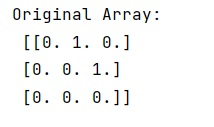# numpy.eye() Method with Example

Python | numpy.eye(): Learn about the numpy.eye() method, its usages and example.
Submitted by Pranit Sharma, on February 27, 2023

NumPy is an abbreviated form of Numerical Python. It is used for different types of scientific operations in python. Numpy is a vast library in python which is used for almost every kind of scientific or mathematical operation. It is itself an array which is a collection of various methods and functions for processing the arrays.

## numpy.eye() Method

The numpy.eye() is used to return a 2-D array with ones on the diagonal and zeros elsewhere. It generates an output in the form of an array where all elements are equal to zero, except for the k-th diagonal, whose values are equal to one.

Syntax:

```numpy.eye(N, M=None, k=0, dtype=<class 'float'>, order='C', *, like=None)
```

Parameter(s):

• N: Number of rows in the output.
• M: Number of columns in the output.
• K: Index of the diagonal: 0 (the default) refers to the main diagonal, a positive value refers to an upper diagonal, and a negative value to a lower diagonal.
• Dtype: Data-type of the returned array.
• order{'C', 'F'}: Whether the output should be stored in row-major (C-style) or column-major (Fortran-style) order in memory.

Let us understand with the help of an example,

## Python code to demonstrate the example of numpy.eye() method

```# Import numpy
import numpy as np

# Creating a numpy image using eye
arr = np.eye(3, k=1)

# Display original image
print("Original Array:\n",arr,"\n")
```

Output:Languages: » C » C++ » C++ STL » Java » Data Structure » C#.Net » Android » Kotlin » SQL
Web Technologies: » PHP » Python » JavaScript » CSS » Ajax » Node.js » Web programming/HTML
Solved programs: » C » C++ » DS » Java » C#
Aptitude que. & ans.: » C » C++ » Java » DBMS
Interview que. & ans.: » C » Embedded C » Java » SEO » HR
CS Subjects: » CS Basics » O.S. » Networks » DBMS » Embedded Systems » Cloud Computing
» Machine learning » CS Organizations » Linux » DOS
More: » Articles » Puzzles » News/Updates